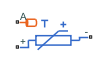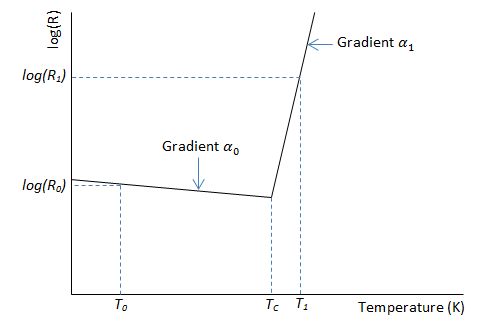# PTC Thermistor

Switching type positive temperature coefficient (PTC) thermistor

• Library:
• Simscape / Electrical / Sensors & Transducers

•## Description

The PTC Thermistor block represents a switching type Positive Temperature Coefficient (PTC) thermistor. This type of thermistor has a decreasing resistance with temperature increasing up to the Curie temperature. Above the Curie temperature the resistance increases very rapidly with increasing temperature, as shown in the following plot. The region to the right of the Curie temperature is called the PTC regime. To represent a non-switching linear PTC thermistor, use the Thermal Resistor block.For a switching type PTC thermistor, the resistance R at temperature T is given by

`${T}_{c}=\frac{\mathrm{log}\left({R}_{1}\right)-\mathrm{log}\left({R}_{0}\right)+{\alpha }_{0}{T}_{0}-{\alpha }_{1}{T}_{1}}{{\alpha }_{0}-{\alpha }_{1}}$`

where:

• Tc is the Curie temperature.

• R0 is the resistance at nominal temperature T0.

• R1 is the resistance at reference temperature T1.

• T0 is the nominal temperature at which the resistance is quoted, usually room temperature. T0 is less than the Curie temperature Tc.

• T1 is the reference temperature, equal or greater than the Curie temperature Tc, which means that at this temperature the PTC regime is in force.

• α0 is the temperature coefficient at nominal temperature T0.

• α1 is the temperature coefficient at reference temperature T1.

The following equation describes the thermal behavior of the block:

`$Q={K}_{d}{t}_{c}\frac{dT}{dt}$`

where:

• Q is the net heat flow into port A.

• Kd is the Dissipation factor parameter value.

• tc is the Thermal time constant parameter value.

• dT/dt is the rate of change of the temperature.

### Variables

To set the priority and initial target values for the block variables prior to simulation, use the Initial Targets section in the block dialog box or Property Inspector. For more information, see Set Priority and Initial Target for Block Variables.

Nominal values provide a way to specify the expected magnitude of a variable in a model. Using system scaling based on nominal values increases the simulation robustness. Nominal values can come from different sources, one of which is the Nominal Values section in the block dialog box or Property Inspector. For more information, see System Scaling by Nominal Values.

## Ports

### Conserving

expand all

Thermal port.

Electrical conserving port associated with the PTC thermistor positive port.

Electrical conserving port associated with the PTC thermistor negative port.

## Parameters

expand all

### Electrical

Nominal resistance of the thermistor at the nominal temperature. Many datasheets quote the nominal resistance at 25°C and list it as R25.

Temperature coefficient at the nominal temperature. The value must be less than zero.

Temperature at which the nominal resistance is measured.

Reference resistance of the thermistor at the reference temperature.

Temperature coefficient at the reference temperature. The value must be greater than zero.

Temperature at which the reference resistance is measured. This temperature must be in the PTC regime.

### Thermal

Time it takes the sensor temperature to reach 63% of the final temperature change when a step change in ambient temperature occurs.

Thermal power required to raise the thermistor temperature by one K.

## Version History

Introduced in R2012b